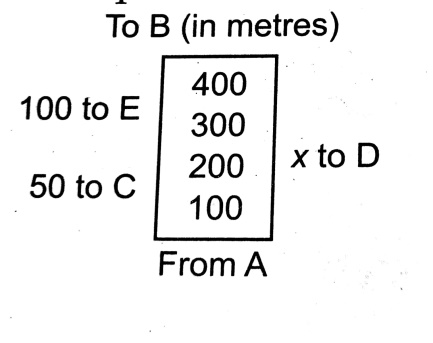# The readings of field book areIt is subsequently realised that the distances to D had been interchanged by mistake. The area of the actual filed would be (a) 1300 sq. m (b) 1500 sq. m (c) 1800 sq. m (d) 2000 sq. m

## Question ID - 50152 :- The readings of field book areIt is subsequently realised that the distances to D had been interchanged by mistake. The area of the actual filed would be (a) 1300 sq. m (b) 1500 sq. m (c) 1800 sq. m (d) 2000 sq. m

3537

Interchanging the distances to C and D, the field may be drawn in th adjoining figure.

We have AB = 40, AF = 30, AG = 20, AH = 10, CH = 30, DG = 20, EF = 30.

Area of the field = ar (AHC) + ar(rect. CEFH) + ar (BEF) + ar (BGD) + ar (AGD)

== 1300 m2.

Next Question :
 The measurements of a filed are as shown.If the total area of the field is 27500 sq. m, then the value of x is equal to (a) 25 m (b) 30 m (c) 50 m (d) 75 m